There are many new and different techniques and approaches in the field of artificial intelligence. One of them is the "Few-Shot Prompting" technique. This technique is a highly effective and easy-to-implement technique for getting the AI model to generate a specific output.

## What is Few-Shot Prompting?

Few-Shot prompting is a highly effective technique for prompting AI models to produce a specific output.

Few-Shot prompting is a technique applied by supporting the model with input-output examples. This technique does not require a large amount of training data and at the output of the prompt, the model gives the desired answers using the training given beforehand.

## Few-Shot Prompting Examples

Let's examine the Few-Shot Prompting technique through the sentiment analysis example and compare it with Zero-Shot Prompting outputs on the same example.

Let's ask the AI model to perform sentiment analysis for the sentence "The food is bad and the service should be improved" using the Zero-Shot Prompting technique (i.e. without any example input-output).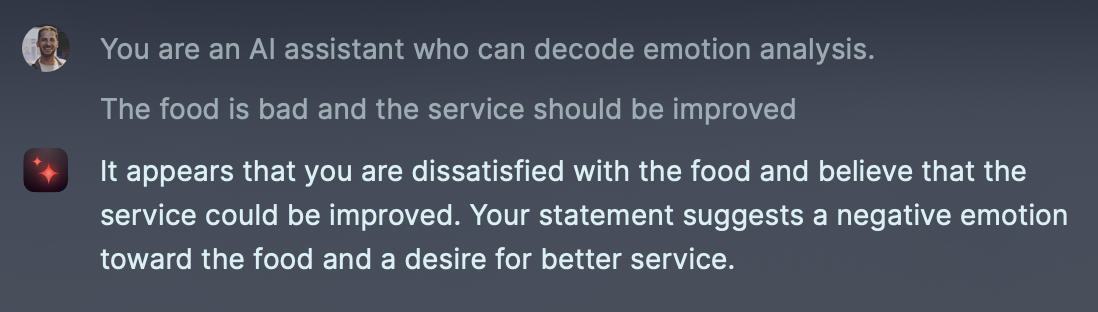As seen here, AI analyzes the sentence and understands that the sentence has a negative structure, but it does not give us the 'negative' answer we want directly, it makes predictions.

Now let's try this task with the Few-Shot Prompting technique.

Let's give the model input and corresponding output examples as follows.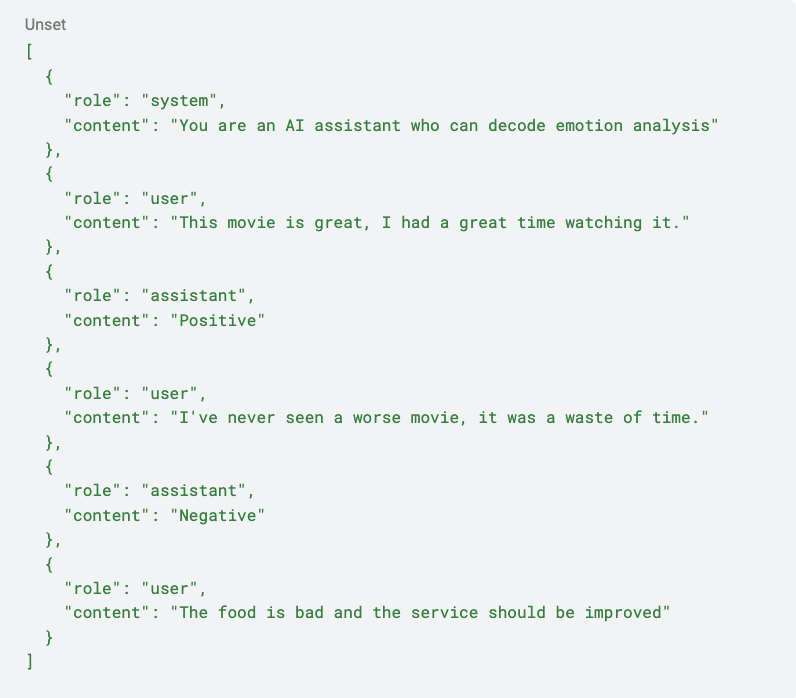With the Few-shot prompting technique, the AI model analyzes the samples before giving the output. As a result of the analysis, the model understands whether the output requested by the user is 'positive' or 'negative'.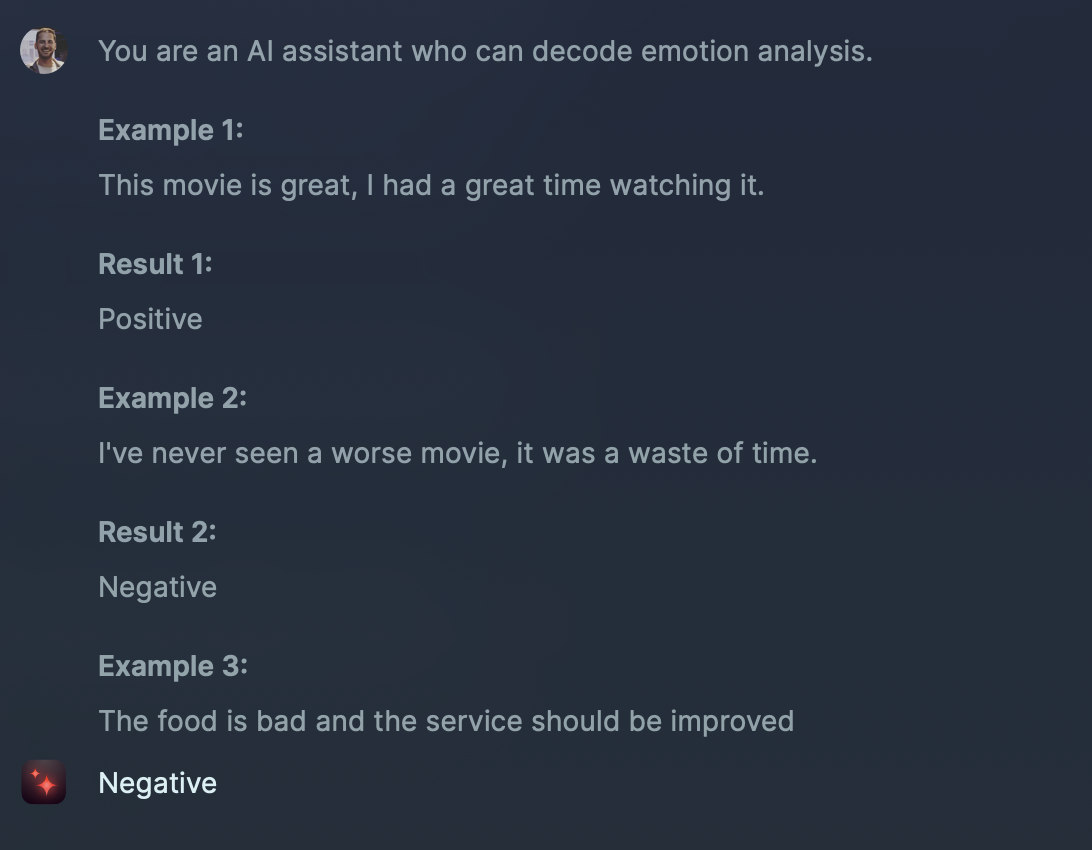The basis of the Few-Shot Prompting technique comes from the tendency of AI models to imitate.

For example, when you give half of a text as input to the AI, the AI tends to continue this text by imitating you and making predictions. So the Few-Shot Prompting technique mimics the input-output examples you give it.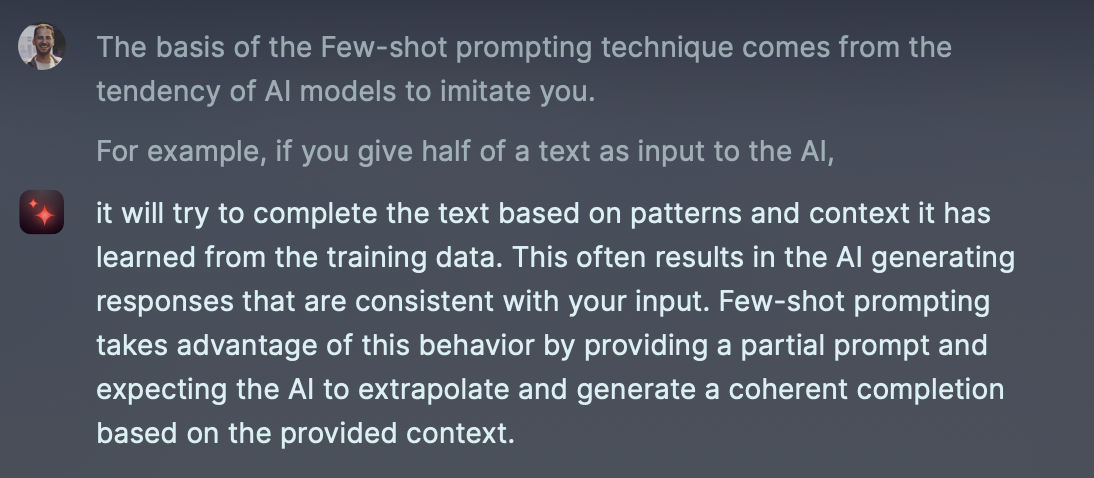## Few-Shot Prompting Limits

Although the Few-Shot Prompting technique can produce highly effective results, it may not be fully successful in specialized or complex problems.

For example, let's imagine that the AI model needs to solve advanced mathematical problems and use the Few-Shot Prompting technique.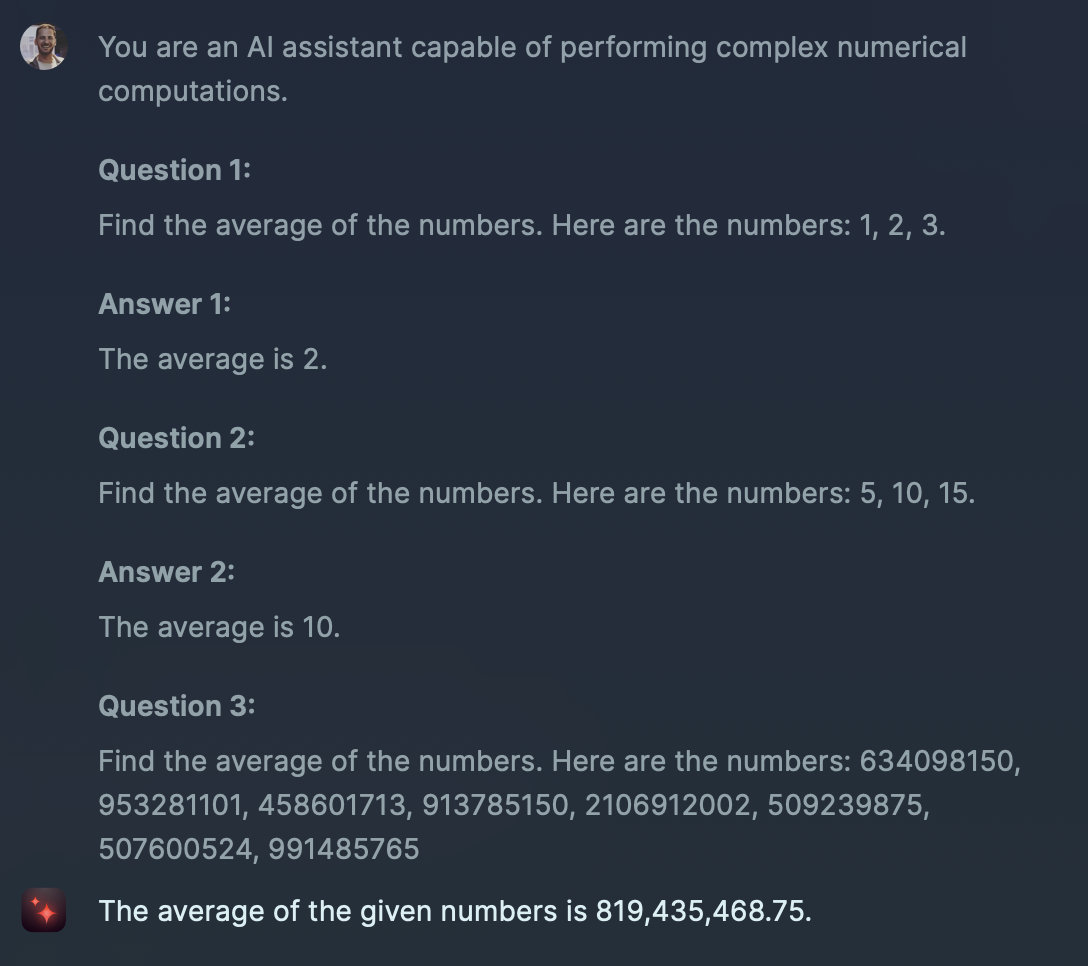As seen in the example, we see that the model gives an erroneous result in a complex and complicated problem. The answer should be 884,375,535.

Note: I strongly recommend using the GPT-4 model in such a complex and complicated problem.

## Tips for Few-Shot Prompting

Tips to consider for improving Few-shot prompts:

### Sample Output Availability

Making sure that the outputs provided in the examples (such as 'positive' and 'negative' for sentiment analysis) are appropriate for your requests will increase the accuracy of the answer.

For example, if you train your model to solve math problems and the outputs in 5 examples are numerical and 1 of them is 'unsolved', this may make it difficult for the model to provide the correct outputs.

### Sample Consistency

Create a consistent format structure in your examples. Giving examples in different formats will make it difficult for the model to give the desired result directly in its answers.

For example, in your Few-Shot prompt you created to solve mathematical problems;

• In the first example, you give '2 plus 2' as input and '4' as output,
• In the second example, suppose you give '3 plus 2' as input and 'This question is an addition operation and the answer is 5' as output.

In the next question, when you want to get the answer directly, as in the first example, the model may try to mimic the output pattern of the second question.

Before giving examples in your prompts, it will make it easier for you to get effective results if you clearly state what you want from the model.

For example, in your Few-Shot prompt for solving mathematical problems, giving the model the task "You are a math professor who can solve difficult problems" will increase the model's effectiveness in understanding the examples given and getting the output you want.

### Example Variety

For the model to generalize its output better, it will be effective to increase the number of examples without taking them out of context.

For example, in your Few-Shot Prompt for solving mathematical problems, giving examples such as addition, subtraction, and square rooting in different but the same context can help you get more accurate outputs in complex questions.

Note: In the Few-Shot Prompting technique, increasing the number of examples too much for the problem can sometimes make the problem more complex. For this reason, I recommend keeping the number of examples at a normal level.

Otherwise, in a Few-Shot Prompting with 50 samples, the model may tend to generate a unique output rather than mimicking your output, and the likelihood of hallucinating increases.

In summary, Few-Shot Prompting is one of the most effective methods to get the output we want from AI models. By creating your Few-Shot prompts from a good strategy framework, we can maximize the outputs we get from AI.